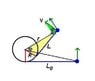# Conservation of angular momentum

• kushan
In summary, the disk experiences force from the string, so its momentum is not conserved. The string is connected to the cylinder. The cylinder is fixed to the table. It stands on the Earth. More or less the system Earth- -table -cylinder -string -disk can be taken a closed system. The velocity is not the same during the motion, as its direction changes.

#### kushan

A horizontal plane supports a stationary cylinder of radius R and a disc A attached to cylinder by a thread of length l , initial velocity given to to disc is v0 .how long will it move until it strikes the cylinder . (no friction)

I guess this question is somewhat like tetherball ,
though i have never seen this game

but I understand the principal and when I try to wrap a particle ( though i performed this with my mobile charger , the bigger part as a the mass and the cable as thread) attached to my finger with a thread ,its velocity increases as it comes closer and closer .
I believe the angular momentum must be conserved .
and my solution book says the tension acting to disc is always perpendicular hence its velocity will always remain constant .
here ,
http://irodovsolutionsmechanics.blogspot.in/2008_05_04_archive.html

Angular momentum is not conserved here, because the force is not central.

how?

Define "central force".

The law of conservation of angular momentum states that when no external torque acts on an object or a closed system of objects, no change of angular momentum can occur.

This isn't a closed system of objects. The cylinder is "fixed".

kushan said:
but I understand the principal and when I try to wrap a particle ( though i performed this with my mobile charger , the bigger part as a the mass and the cable as thread) attached to my finger with a thread ,its velocity increases as it comes closer and closer .
I believe the angular momentum must be conserved .
and my solution book says the tension acting to disc is always perpendicular hence its velocity will always remain constant .

I repeated your experiment with the mobile charger. How could you be sure that the speed of your mobile charger increased? The angular speed evidently did increase but it can happen with constant linear speed.
The velocity has to be perpendicular to the thread. The disk can not stretch out the thread and if it had an inward parallel component the thread would get loose.
Force times velocity is power, work per unit time. If they are perpendicular, no work is done. No work, no change of kinetic energy.

ehild

There is an easier solution than the one in the book. See attachment. Think in polar coordinates. Draw the radius at a time instant from the centre of the cylinder to the small disk (r) and the other radius tangent to the thread (R). The radii make angle φ. The velocity V also encloses the angle φ with radius r. Decompose the velocity into radial and azimuthal components. Vr=dr/dt=-Vcos(φ). From the yellow triangle, cosφ=R/r, and r2=L2+R2.

Eliminating cos(φ), you get the equation dr/dt=-VR/r---->rdr/dt=-VR ---->(1/2)d(r2)/dt=-VR. When the disc hits the cylinder r=R. Integrate the left side with respect to t from t=0 to t=T, the right side with respect to r2, from L02+R2 to R2.

ehild

#### Attachments

•wrapping.JPG
8.1 KB · Views: 344
Thanks everyone .
I really found polar method simple .
Thanks for the diagram ehild .
I got the answer perfect .
But when to conserve momentum and when not to .
The definition says when no external force .
But here also no external force .
Then why same velocity

kushan said:
But here also no external force .

As I already said, this is not a closed system. The cylinder is "stationary", which means there are forces acting on it to keep it stationary. If you had just the cylinder and the disk connected by a string somewhere in the outer space, then there would be conservation of angular momentum, but the motion would be different.

kushan said:
But when to conserve momentum and when not to .
The definition says when no external force .
But here also no external force .
Then why same velocity

The disk experiences force from the string, so its momentum is not conserved. The string is connected to the cylinder. The cylinder is fixed to the table. It stands on the Earth. More or less the the system Earth- -table -cylinder -string -disk can be taken a closed system.
The velocity is not the same during the motion, as its direction changes.

The string exerts force on the disk. This force has torque with respect to the fixed axes of the cylinder. So the angular momentum of the disk also changes.

The kinetic energy is conserved as the force of the string is perpendicular to the velocity of the disk. Therefore the speed is constant.

ehild